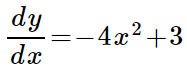### Day 54 - Antiderivative - 04.06.15

Update
• Unit 3 Test
• Average = 81%

Questions

Bell Ringer
1. What is the function that has a derivative of f’(x) = 2?

1. f(x) = 2x

2. f(x) = x2

3. f(x) = 2x + 1

4. f(x) = 2x - 3

5. more than one answer exists

2. Which of the following functions have a derivative of f’(x) = 7x and f(0) = 7

1. f(x) = x2 + 7

2. f(x) = (7/2)x2 + 8

3. f(x) = (7/2)x2 + 7

4. f(x) = -(7/2)x2 - 7

5. none of the above

3. Which of the following functions have a second derivative of f”(x) = 5

1. f(x) = 5x

2. f(x) = (5/2)x2 + 4

3. f(x) = (5/2)x2 - 4

4. more than one answer exists

5. none of the above

4. Solve for y:1. y = (-4/3)x3 + 3x + C

2. y = (4/3)x3 + 3x + C

3. y = (-4/3)x3 - 3x + C

4. y = (4/3)x3 - 3x + C

5. none of the above

Review
• Prerequisites
• Derivatives
• Derivative Rules
• Power Rule
• Constant Rule
• Trig Derivatives

Lesson
• If the derivative of a function is f'(x) = 3, what is the function?
• Antiderivative (video)
• Indefinite Integral
• Definition
• Integral Sign
• Integrand
• Variable of Integration
• Constant of Integration
• General Solution
• Basic Integration Rules

Exit Ticket
• Posted on the board at the end of the block
Lesson Objective(s)
• How can the antiderivative of a function be calculated?
Skills
1. Calculate the antiderivative of a function.

#### In-Class Help Requests

Standard(s)
• APC.10
• Use Riemann sums and the Trapezoidal Rule to approximate definite integrals of functions represented algebraically, graphically, and by a table of values and will interpret the definite integral as the accumulated rate of change of a quantity over an interval interpreted as the change of the quantity over the interval
• Riemann sums will use left, right, and midpoint evaluation points over equal subdivisions.
• APC.11
• ​The student will find antiderivatives directly from derivatives of basic functions and by substitution of variables (including change of limits for definite integrals).
• APC.12
• ​The student will identify the properties of the definite integral. This will include additivity and linearity, the definite integral as an area, and the definite integral as a limit of a sum as well as the fundamental theorem.
• APC.13
• ​The student will use the Fundamental Theorem of Calculus to evaluate definite integrals, represent a particular antiderivative, and facilitate the analytical and graphical analysis of functions so defined.
• APC.14
• ​The student will find specific antiderivatives, using initial conditions (including applications to motion along a line). Separable differential equations will be solved and used in modeling (in particular, the equation y' = ky and exponential growth).
• APC.15
• ​The student will use integration techniques and appropriate integrals to model physical, biological, and economic situations. The emphasis will be on using the integral of a rate of change to give accumulated change or on using the method of setting up an approximating Riemann sum and representing its limit as a definite integral. Specific applications will include
• a)​ the area of a region;
• b)​ the volume of a solid with known cross-section;
• c)​ the average value of a function; and
• d) ​the distance traveled by a particle along a line.

Past Checkpoints
• N/A